##### Actions

(diff) ← Older revision | Latest revision (diff) | Newer revision → (diff)

In its basic form, the study of the interplay between an embedding of a projective manifold (cf. Projective algebraic set)into projective space and its canonical bundle,, whereis the cotangent bundle of. For simplicity, below the objects are considered over the complex numbers. The book [a1] is a general reference with full coverage of the literature for the whole theory with its history. The book [a4] is a fine reference on related material.

To study a smooth projective algebraic curve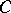, i.e., a compact Riemann surface, a major approach in the 19th century was to relate properties of the curve to properties of the canonical mapping of the curve, i.e., the mapping of the curve into projective space given by sections of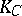. To study a two-dimensional algebraic submanifold of projective space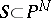, it was natural to try to reduce questions about the surfaceto the hyperplane sections of, i.e., to the curves obtained by slicingwith linear hyperplanes. This led to the study of the adjoint bundle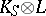, whereis the restriction toof the hyperplane section bundle of, i.e., the line bundle on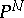whose sections vanish on hyperplanes of. The restriction of the adjoint bundle to a hyperplane sectionofis the canonical bundle of, and, except in a few trivial cases,is the only line bundle that has this property. Therefore, if the mapping associated toexists, it would tie together the canonical mappings of the smooth hyperplane sections of. In the 19th century geometers, especially G. Castelnuovo and F. Enriques, used this rational mapping to study the embedding ofinto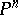. The general procedure was to consider the sequence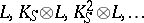. A classical result was that adjunction terminates, i.e., there is a positivesuch thathas no sections, if and only ifis birational (cf. Birational morphism) to the product of an algebraic curve and. A key point was that if the abovewas the first positivesuch thathas no sections, then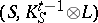is one of only a "very short list of pairs" . Classically, it was not known ifwas spanned and, therefore, this procedure did not usually lead to a biregular classification. (A line bundleon an algebraic set is said to be spanned if global sections ofsurject onto the fibre of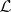at any point of the algebraic set.) There were also analogous classical procedures on threefolds due to G. Fano and U. Morin (see [a5], [a1]).

A.J. Sommese [a6] started the modern study of the mapping (which he called the adjunction mapping) associated to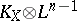, where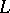is a very ample line bundle (cf. Ample vector bundle) on an-dimensional projective manifold. The complete story with history of the adjunction mapping can be found in [a1], Chapts. 8–11. The fundamental results of this theory are that except for a few special varieties, the pair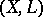can be replaced by a closely related pair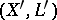with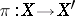the blow-up of an-dimensional projective manifold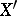at a finite set,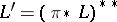an ample line bundle,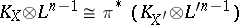, and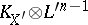very ample. Moreover, ifthen, except for a few more examples,is numerically effective, or nef, i.e.,for any effective curve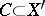. These results allow the classical birational results alluded to above to be both considerably extended and redone biregularly.

The major open question for this part of adjunction theory is to what extent the mapping associated to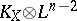is well-behaved whenis very ample,is smooth, and. For example, it is known whenthat, except for obvious counterexamples,, and if the Kodaira dimension ofis non-negative, thenunless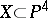is a quintic threefold withtrivial. See [a2], [a1] for this and some further discussion of this problem.

A more abstract approach to adjunction theory is to start with a pairwithan ample line bundle on a projective varietyhaving at worst mild singularities, e.g., terminal singularities. Then, assuming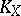is not nef, Kawamata's theorem asserts that there is a rational number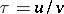with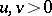, called the nefvalue of the pair such thatis nef but not ample. By the Kawamata–Shokurov theorem,is spanned for all sufficiently large positive integers. The morphism, fromto a normal projective variety, associated to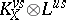, is independent offor all sufficiently large. This mapping, called the nefvalue morphism of the pair, has connected fibres, and at least one positive-dimensional fibre. If, one can expressas a very special fibration of Fano varieties, e.g., if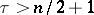, thenis a contraction of an extremal ray (see [a3] and [a1], Chapt. 6). If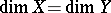, then one can replacewith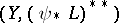and repeat the procedure. This works well for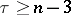(see [a1], Chapts. 6, 7).
Define the spectral valueof the pairas the infimum of the positive rational numberswithsuch that there is some positive integer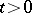with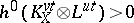. A major conjecture in this part of the theory is that if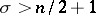, thenis the nefvalue of the pairand the nefvalue morphism has a lower-dimensional image.